# Understanding Proportionality through Visualization

## Introduction

In this vignette, we use a real dataset to show how to apply proportionality analysis to RNA-seq count data. We place a particular emphasis here on documenting $$\rho$$ and the visualization tools included with this package. Although the user may feel eager to start here, we strongly recommend first reading the companion vignette, “An Introduction to Proportionality”.

## Sample data

As a use case, we analyze raw RNA-seq counts from a published study on cane toad evolution and adaptation (Rollins 2015). This dataset contains the transcript counts for 20 toads, sampled from two locations in the wild. Sugar cane farmers introduced cane toads to Australia in 1935 as a pest control measure, but these toads quickly became pests themselves. Starting in Queensland (QLD), they have since spread out into parts of Western Australia (WA). The two locations sampled, which we will treat as the experimental groups, include the early settlement region in QLD and the front of expansion into WA. In this analysis, we try to tease apart the genomic differences between the regional and invasive toads. To begin, we load the propr library along with the example data.

library(propr)
data(caneToad.groups)

## Proportionality analysis

Next, we calculate proportionality between all transcripts in the dataset. When working with a large number of transcripts (57,580 in this case), we may first wish to filter non-informative transcripts to minimize the computational burden of analysis. However, while the numerator portion of $$\rho$$ remains fixed regardless of pre-filtering, the denominator portion of $$\rho$$ does change. In other words, although the numerator portion is sub-compositionally coherent, the denominator portion is not. Therefore, pre-filtering before calculating $$\rho$$ impacts the final result.

In some circumstances, it is simply infeasible to calculate a proportionality matrix using all features. For example, calculating pairwise proportionality here requires at least 32 GB of RAM, not counting the additional RAM required for indexing and visualization. However, beginning with version 2.0.3, we can now pre-filter while calculating $$\rho$$, which does not impact the final result. For this pre-filter step, we remove all transcripts that do not have at least 10 counts in at least 10 samples. We base this on the intuition that lowly-expressed transcripts likely have high relative error in cross-sample comparisons due to random noise from the assay technology and alignment protocol. Note that beginning with version 2.2.0, caneToad.counts is bundled as already pre-filtered in order to satisfy strict file size limits imposed by CRAN.

keep <- apply(caneToad.counts, 2, function(x) sum(x >= 10) >= 10)
rho <- perb(caneToad.counts, select = keep)

Next, we index the most highly proportional pairs based on an arbitrary threshold. In the absence of any statistical testing framework, we might set this threshold at $$\rho>0.95$$ to include only “very proportional” transcript pairs. Alternatively, we could set this threshold at $$\rho<-0.95$$ to include only “very unproportional” pairs. Take note that we use a more stringent threshold here so that the vignette renders more quickly. For more information on selecting a proportionality cutoff, we refer the reader to the “How Proportional Is Proportional Enough?” vignette.

best <- rho[">", .995]

## Index-aware plots

After indexing, it is helpful to check the pairwise distribution of proportional pairs using the plot (or, equivalently, smear) function. As an “index-aware” function, plot only plots feature pairs indexed with [. When interpreting this figure, a smear of straight diagonal lines confirms that the pairs are proportional. Intuitively, this means that as one transcript increases in log-ratio transformed abundance, so does the other. If you see lines deviating considerably from the $$y=x$$ diagonal, you likely set your index threshold too low.

plot(best)
## Alert: Generating plot using indexed feature pairs.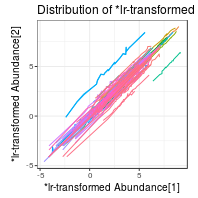You can also inspect how the indexed pairs cluster using the dendrogram function. Specifically, this function clusters features based on a hierarchical clustering of the proportionality matrix. In this package, we define the dissimilarity measure as as.dist(1-abs(rho@matrix)). Like plot, this function is “index-aware” and only plots feature pairs indexed with [. Take note that this dendrogram matches the dendrogram used to label co-clusters in the prism, bokeh, and bucket plots below, but differs from the dendrogram produced by the snapshot plot. Heatmap intensity is not scaled.

dendrogram(best)
## Alert: Generating plot using indexed feature pairs.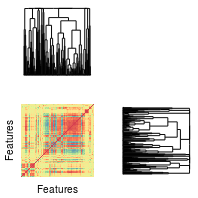## Index-naive plots

The remaining visualization methods do not restrict plotting to indexed pairs, but instead incorporate all features in the propr object. To exclude features that do not belong to an indexed feature pair, we simplify the indexed propr object.

best <- simplify(best)

Compositional data are not distributed in Euclidean space, but rather exist in a space known as the Aitchison simplex (Fernandes 2014). The log-ratio transformation transforms such data to Euclidean space, allowing us to summarize our data through conventional statistics.

First, we look at pca, a function for visualizing the samples across the first two dimensions as reduced by principal components analysis (PCA). This is a valid implementation of PCA for relative data, computed using log-ratio transformed data (Gloor 2016). The group argument allows us to color the sample IDs by group membership.

pca(best, group = caneToad.groups)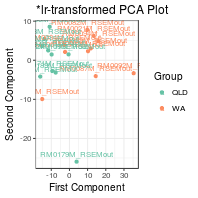Second, we look at snapshot, a function for visualizing the intensity of the log-ratio transformed data across samples. Heatmap intensity is not scaled.

snapshot(best)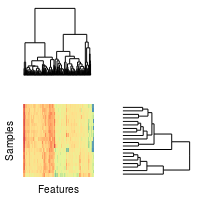Finally, we look at prism, bokeh, and bucket (pronounced bouquet), three functions for visualizing the co-clustering of proportional features. We mention these plots together because they share some key similarities. First, they are “index-naive” and plot all $$\rho$$ in the @matrix slot of the propr object. Second, they identify the feature pairs where both constituents co-cluster (with the total number of clusters toggled by k). Third, they return a vector of cluster memberships for all features in the propr object.

The prism function plots the variance of the ratio of the log-ratio transformed feature pair (VLR) versus the sum of the individual variances of each log-ratio transformed feature (VLS). The ratio of the VLR to the VLS equals $$1 - \rho$$. As such, we use here seven rainbow colored lines to indicate where $$\rho = [.01, .05, .50, 0, 1.50, 1.95, 1.99]$$, going from red to violet. A low VLR with a high VLS suggests that the feature pair remains in an equilibrium despite high variability among the individual features.

clusts <- prism(best, k = 5)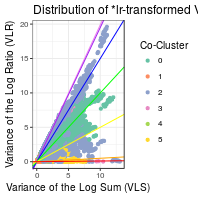The bokeh function plots pairs across the individual variances of the constituent log-ratio transformed features. For clarity of visualization, this figure projects the data on a log-fold scale. Therefore, the highly variable co-clusters appear in the top-right of the figure while the lowly variable co-clusters appear in the bottom-left. Meanwhile, highly proportional pairs tend to aggregate around the $$y=x$$ diagonal. The user can retrieve the table used to generate the prism and bokeh plots by passing the propr object through the slate function.

clusts <- bokeh(best, k = 5)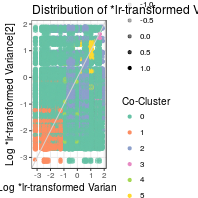The bucket function plots an estimation of the degree to which a feature pair differentiates the experimental groups versus the proportionality between that pair.

clusts <- bucket(best, group = caneToad.groups, k = 5)
## Warning: Removed 1 rows containing missing values (geom_hline).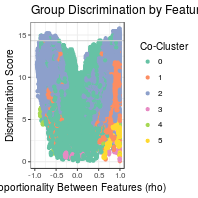These plots can help us conceptualize high-dimensional data and select a highly proportional module for further analysis. In this example, we choose co-cluster 2 for further analysis because it shows high proportionality in the setting of high individual feature variance.

## Down-stream

We can extract co-cluster 2 from the propr object using the subset method.

sub <- subset(best, select = (clusts == 2))
## Alert: User must repopulate @pairs slot after subset.

We can visualize this cluster as a network using the cytescape function. The resultant figure shows indexed proportional pairs as edges connecting the constituent features. Providing feature names to the optional arguments col1 and col2 will color those nodes red and blue, respectively. We recommend using this function for integrating proportionality analysis with conventional differential expression analysis. Note that setting d3 = TRUE will have the rgl package render the network in 3D.

cyt <- cytescape(sub[">", .95])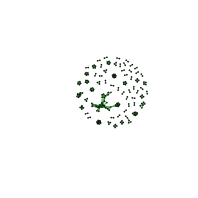We can use the pca function to see how well this cluster differentiates the two experimental groups. We see here that the first two principal components of this highly proportional module leads good separation between the experimental groups. This matches the separation achieved in the source publication which used edgeR for feature selection (Rollins 2015), suggesting that our unsupervised method identified a feature subset that is relevant to the experimental condition. Note, however, that our approach prioritizes features with known coordination across all samples.

pca(sub, group = caneToad.groups)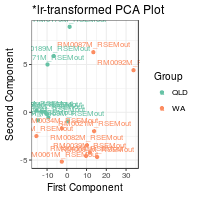Having identified a cluster that separates the experimental groups, the next step in this pipeline might involve a gene set enrichment analysis (GSEA) of the transcripts participating in this highly proportional module. The application of GSEA is beyond the scope of this vignette, although we can easily extract the names of the transcripts that belong to this cluster.

transcripts <- colnames(sub@logratio)

## Conclusions

We introduce here a simple tool that is valid for all biological count data. In the example above, we show how this package can uncover a highly proportional transcript module that differentiates the experimental groups. We find this feat particularly impressive considering that, with the exception of the superfluous bucket plot, we discovered this module without ever specifying how the experimental groups should guide feature selection. We believe that this unsupervised application of proportionality analysis could offer unique insights into biological systems.